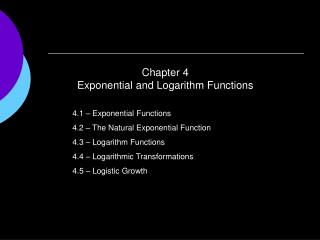DownloadDownload PresentationChapter 4 Exponential and Logarithm Functions

# Chapter 4 Exponential and Logarithm Functions

Télécharger la présentation## Chapter 4 Exponential and Logarithm Functions

- - - - - - - - - - - - - - - - - - - - - - - - - - - E N D - - - - - - - - - - - - - - - - - - - - - - - - - - -
##### Presentation Transcript

1. Chapter 4Exponential and Logarithm Functions 4.1 – Exponential Functions 4.2 – The Natural Exponential Function 4.3 – Logarithm Functions 4.4 – Logarithmic Transformations 4.5 – Logistic Growth

2. Section 4.1Exponential Functions Review of Laws of Exponents (p157 & 158) Characteristics of Exponential Functions Modeling with Exponential Functions

3. Basic Exponential Functions

4. Basic Exponential Functions: f(x) = 4x f(x) = 4x domain: reals range: positive reals y intercept: 1 increasing a > 1

5. Basic Exponential Functions: f(x) = 4x as x increases without bound, 4x increases without bound as x decreases without bound, 4x gets close to 0 as x → ∞, 4x → ∞ as x → -∞, 4x → 0 y = 0 is a Horizontal Asymptote

6. Basic Exponential Functions: f(x) = (¼)x f(x) = (¼)x domain: reals range: positive reals y intercept: 1 decreasing 0 < a < 1

7. Basic Exponential Functions: f(x) = (¼)x as x increases without bound, (¼)x gets close to 0. as x decreases without bound, (¼)x increases without bound as x → ∞, (¼)x → 0y = 0 is a Horizontal Asymptote as x → -∞, (¼)x → ∞

8. Basic Exponential Functions f(x) = 4x and g(x) = (¼)x graphs are reflections about the y axis Does f(-x) = g(x)?

9. Basic Exponential Functions f(x) = (a)x and f(x) = c*ax f(x) = 5*4x domain: reals range: positive reals HA: y = 0 y intercept: 5 f(x) = 4x domain: reals range: positive reals HA: y = 0 y intercept: 1

10. Basic Exponential Functions: f(x) = c*ax Characteristics f(x) = c*ax domain: reals range: positive reals HA: y = 0 y intercept: c increasing for a > 1decreasing for 0 < a < 1

11. Variations of Basic Exponential Functions f(x) = 5*4x domain: reals range: positive reals HA: y = 0 y intercept: 5 f(x) = 20 + 5*4x domain: reals range: reals > 20 HA: y = 20 y intercept: 25

12. Modeling with Exponential Functions Example/156 A math student pours himself a mug of steaming coffee and then forgets to drink it. In a room that remains at 20C, the coffee cools, losing heat rapidly at first and then more slowly as the liquid approaches room temperature. The coffee is initially 90C and after 10 minutes cools to 68C. Find a model for the temperature of the coffee over time. exponential with 0 < a < 1 and shifted up 20 units H(t) = V + c*at

13. Modeling with Exponential Functions H(t) = V + c*at Can we solve for V, c, and a? V = 20 so H(t) = 20 + c*at Vertical shift H(0) = 9020 + c*a0 = 9020+c = 90c = 70 so H(t) = 20 + 70*at Initial Temperature H(10) = 6820 + 70*a10 = 6870*a10 = 48a10 = 48/70a = (48/70)(1/10)a = 0.963 so H(t) = 20 + 70*(0.963)t Another data point

14. Modeling with Exponential Functions Example/156 A gymnastics team practices its balance-beam routine, improving month by month. Initially the average score is 3.8 but then scores increase rapidly; as more time passes, additional efforts result in small gains. In fact, after 6 months of practice, the average score is 5.7 Find a model for the average score of the team over time. exponential with 0 < a < 1, reflected about x axis and shifted up. S(t) = V - c*at

15. Modeling with Exponential Functions S(t) = V - c*at Can we solve for V, c, and a? V = 10 so S(t) = 10 - c*at Vertical shift S(0) = 3.810 - c*a0 = 3.810 - c = 3.8c = 6.2 so S(t) = 10 – 6.2*at Initial Score S(6) = 5.710 – 6.2*a6 = 5.7-6.2*a6 = -4.3a6 = -4.3/-6.2a = (4.3/6.2)(1/6)a = 0.94 so S(t) = 10 – 6.2*(0.94)t Another data point

16. Section 4.2The Natural Exponential Function What is e? Base-e Exponential Functions

17. Natural Exponential Function: f(x) = ex = (2.718)x f(x) = ex domain: reals range: positive reals y intercept: 1 increasing a > 1

18. Homework Pages 193-194 #1-16 Turn In: #7,#8, #13